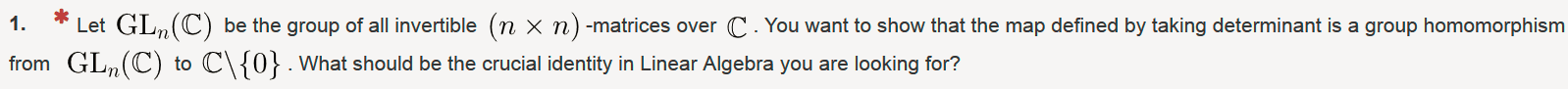# Connecting linear algebra concepts to groups

• MHB
lemonthreeThe options are
$$\displaystyle rank(B)+null(B)=n$$
$$\displaystyle tr(ABA^{−1})=tr(B)$$
$$\displaystyle det(AB)=det(A)det(B)$$

I'm thinking that since it's invertible, I would focus on the determinant =/= 0. I believe the first option is out, because null (B) would be 0 which won't be helpful. The second option makes the point that $$\displaystyle AA^{−1}$$ is $$\displaystyle I$$, so it's suggesting invertibility. So I'm deciding between the second and last option. Does anyone have any tips?

Last edited by a moderator:

HOI
Since the problem specifically refers to "the map defined by taking determinants", I would almost automatically check "det(AB)= det(A)det(B)"! "Almost" because, of course, I would want to make sure it was correct.

lemonthree
Since the problem specifically refers to "the map defined by taking determinants", I would almost automatically check "det(AB)= det(A)det(B)"! "Almost" because, of course, I would want to make sure it was correct.

Agree, I was tempted to select det(AB)= det(A)det(B) but then again I can't be fully sure.

I guess since we both believe it to be correct, I guess I'm going for this answer then!

HOI
I didn't say I believe it to be correct! I said I would "almost" automatically check it. And I said "of course, I would want to make sure it was correct". Why can't you "be fully sure"?

lemonthree
I didn't say I believe it to be correct! I said I would "almost" automatically check it. And I said "of course, I would want to make sure it was correct". Why can't you "be fully sure"?

Good point! My gut feeling just told me that the determinant is the answer but my brain told me to be careful.

Homework Helper
MHB
Let's take a closer look, and let's start with the crucial bit of information that we need: what is the definition of a group homomorphism?

lemonthree
Hi Klaas, indeed, it is det(AB) = det(A)det(B) because as per the definition of group homomorphism, φ (G) = H if φ (xy) = φ (x) φ (y)